# Graph Plotting in Python Using Matplotlib

In Python, the Matplotlib library plots various graphs using the input data. Matplotlib library is a visualization utility library. It provides various plots such as Bar charts, histograms, Piechart, scatter plots, etc. Let’s discuss each in detail.

## Graph Plotting in Python Using Matplotlib

Before plotting any graph using the Matplotlib library, install the module matplotlib using the below command.

`pip install matplotlib`

Below is the simple line plot using the matplotlib library.

```# import matplotlib module
import matplotlib.pyplot as plt

# Data points
x = [0,1,2]
y = [0,1,2]

# plotting the x,y coordinates
plt.plot(x, y)

# naming the axis
plt.xlabel('X-axis')
plt.ylabel('Y-axis')

# Specifying title
plt.title('Line Plot')

# shows the plot
plt.show()```

Output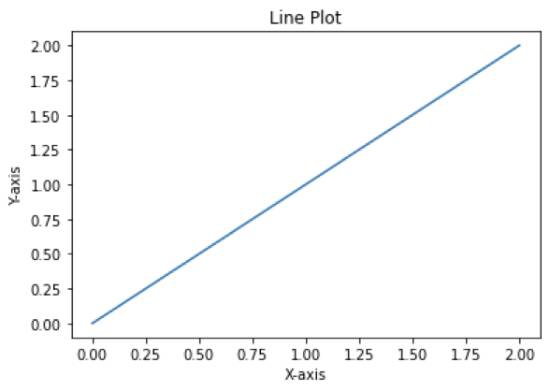Code Explanation:

• Import required modules/libraries.
• Plot the data points using the plot() function.
• Name the x-axis & y-axis using the xlabel() & ylabel() methods.
• The plot’s title can be given using the title() function.
• To view the plot, use the show() method.## Plot Multiples lines in a single chart

Code:

```# import matplotlib module
import matplotlib.pyplot as plt

# Data points
x1 = [0,1,2]
y1 = [0,1,2]

x2 = [0,1,2]
y2 = [2,1,0]

# plotting the 2 lines
plt.plot(x1, y1, label='Line-1')
plt.plot(x2, y2, label='Line-2')

# naming the axis
plt.xlabel('X-axis')
plt.ylabel('Y-axis')

# Specifying title
plt.title('Multiple Lines Plot')

# shows the plot
plt.legend()
plt.show()```

Output: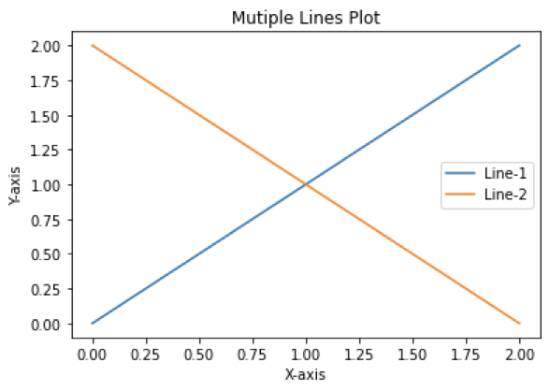Also ReadHow to Create a Neural Network in Python

Explanation:

• When multiple lines are plotted in a graph, we can give a name to each line by passing a label argument to the plot() function to differentiate the lines.
• The legend() function gives information about the name (label) of the line and its color.

Some customizations can be made to a line plot by changing the style of the line, the width of line, color of line, marker point, size, color, etc. Below is the code which plots a line plot using a few customized options.

Code:

```# import matplotlib module
import matplotlib.pyplot as plt

# Data points
x = [0,1,2]
y = [0,1,2]

# plotting the x,y coordinates
plt.plot(x, y, linestyle='dashed', color='red', linewidth = 4, marker='*', markerfacecolor='Black', markersize=20)

# naming the axis
plt.xlabel('X-axis')
plt.ylabel('Y-axis')

# Specifying title
plt.title('Customized Line Plot')

# shows the plot
plt.show()```

Output: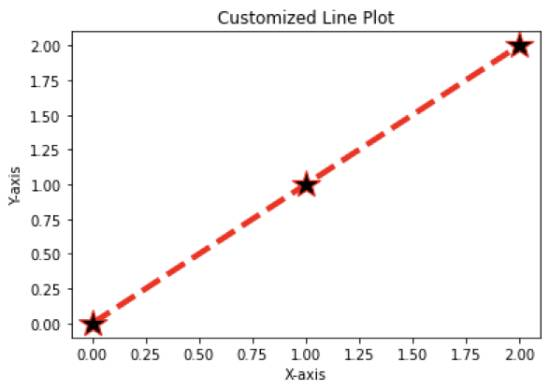## Scatter plot using matplotlib

In the Scatter plot, each data point is represented as a dot. The scatter() method is used to plot the scatter plot, and the marker point will default be a dot. The marker can be changed by passing the marker argument into the scatter method.

Syntax: `matplotlib.pyplot.scatter(x, y, marker, color)`

Below is the simple code of scatter plot using the matplotlib library.

Code:

```# import matplotlib module
import matplotlib.pyplot as plt

# Data points
x = [0,1,2]
y = [0,1,2]

# scatter plot
plt.scatter(x, y, marker='*',color='red')

# naming the axis
plt.xlabel('X-axis')
plt.ylabel('Y-axis')

# Specifying title
plt.title('Scatter Plot')

# shows the plot
plt.show()```

Output: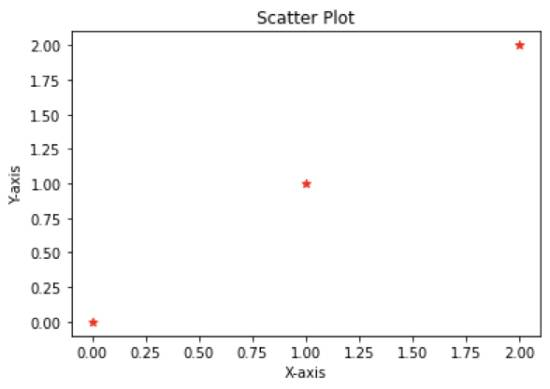## Bar plot using matplotlib

The bar() method plots the Bar chart in Python using the matplotlib library. The bar() method accepts parameters of x, height of bars, color of bars, width of bars, etc. The syntax of the bar() method is given as follows –

`matplotlib.pyplot.bar(x, height, width, color)`

The below code plots a bar chart representing the runs scored by different players.

Code:

```# import matplotlib library
import matplotlib.pyplot as plt

# data
Player = ['Virat', 'Mahesh Babu', 'Rohit', 'ABD']
Runs = [100, 71, 58, 120]

# plotting a bar chart
plt.bar(Player, Runs, color = ['Blue', 'Black', 'Green', 'Red'])

# naming the axis
plt.xlabel('Players')
plt.ylabel('Runs')

# Specifying title
plt.title('Runs scored by players')

# show the plot
plt.show()```

Output:Also ReadHow To Copy List in Python

## Plotting a Histogram using the Matplotlib library

A histogram represents the given data in the form of groups. In Python, the Matplotlib library provides the hist() method, which accepts data and groups the data based on specified bins.

Syntax: `matplotlib.pyplot.hist(data, bins, range, histtype)`

Parameters:

• Data: Input data.
• Bins: Specify the number of bins.
• Range: Represents the lower limit and upper limit.
• Histtype: Specifies the type of histogram (bar, step)

Code:

```# import matplotlib module
import matplotlib.pyplot as plt

# Marks of students
marks = [100,99,80,34,9,39,90,83,55,57,76,43,72,44,50]

# bin count
bins=10
range=(0,100)

# plotting a histogram
plt.hist(marks, bins, range, histtype='bar', rwidth=0.5)

# naming the axis
plt.xlabel('Marks')
plt.ylabel('Count')

# Specifying title
plt.title('Histogram')

# shows the plot
plt.show()```

Also Read10 Best Programming Apps To Learn Python

Output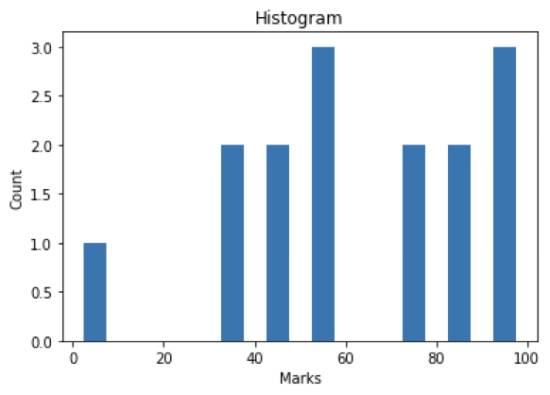Also ReadPython Program To Reverse A Number

## Curve plotting in matplotlib

Curve plotting can be done using the plot() function as same as a simple line plot, but data used to plot will be generated using the sin() and cos() functions.

Code:

```# import required libraries
import matplotlib.pyplot as plt
import numpy as np

data1 = np.arange(0, np.pi, 0.1)
data2 = np.sin(data1)

plt.plot(data1, data2)

# naming the axis
plt.xlabel('X-Axis')
plt.ylabel('Y-Axis')

# Specifying title
plt.title('Curve plot')

# shows the plot
plt.show()```

Output: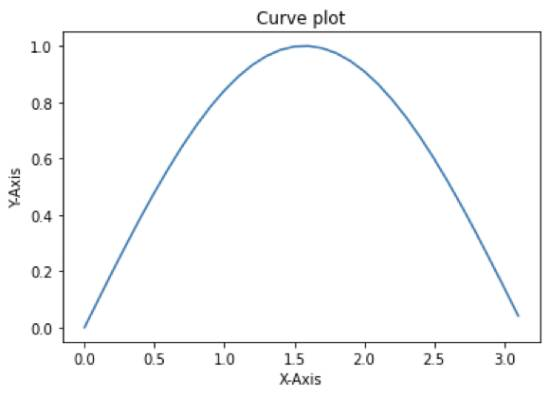## Pie chart

The Pie chart displays a circular statistical plot of the given data. To plot the pie chart, Python provides a method called pie() which accepts a data series.

Syntax: `matplotlib.pyplot.pie(data, labels)`

Below is a simple pie chart that shows the percentage of motor vehicles (Two wheelers) of different brands sold in a year.

```# import necessary libraries
import matplotlib.pyplot as plt
import numpy as np

Sales = np.array([40, 30, 20, 5, 5])
Company = ["Hero", "Honda", "Yamaha", "Bajaj", "TVS"]

plt.pie(Sales, labels = Company)
plt.show()```

Output: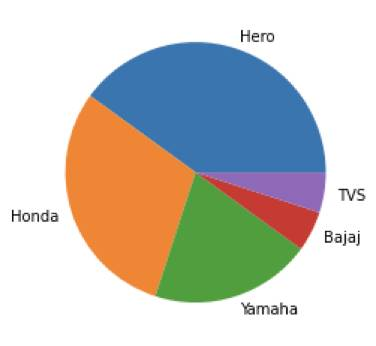### Summary

In this tutorial, we explored graph plotting in Python. We shared how to plot various graphs in Python, such as line charts, bar graphs, and histograms. Subscribe to our newsletter to get similar articles straight to your inbox.

Scroll to Top